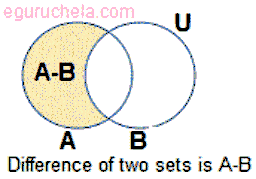# Difference of Two Sets Calculation

The set value to be entered with comma seperated example : 2,3,5,8,10

 Enter the elements of Universal set(A): Enter the elements of the set(B):

Set Difference A - B :The difference of set B in set A is the complement of B in A.

If A and B are any two sets, then the relative complement of B in A is the set of all elements in A which are not in B.

It is denoted by A - B. Difference of two sets A and B, A - B is a set whose elements belong to A but not to B.

A - B is also called the relative complement of B

### Example

Find A – B and B – A for given values :

A = {2, 3, 5, 8, 10, 15} and

B = {7, 8, 10, 12, 13}.

#### Solution:

The given values,

A = {2, 3, 5, 8, 10, 15}

B = {7, 8, 10, 12, 13}

A – B = {2, 3, 5, 15} since the elements 2, 3, 5, 15 are there in A but not in B.

Similarly,

B – A = {7,12,13}, since the elements 7, 12, 13 belong to B and not to A.

Therefore, we can say that A – B ≠ B – A.